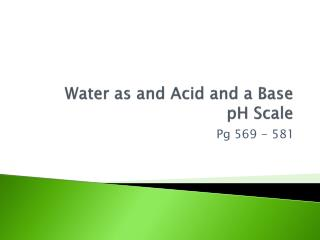DownloadDownload PresentationWater as and Acid and a Base pH Scale

# Water as and Acid and a Base pH Scale

Download Presentation## Water as and Acid and a Base pH Scale

- - - - - - - - - - - - - - - - - - - - - - - - - - - E N D - - - - - - - - - - - - - - - - - - - - - - - - - - -
##### Presentation Transcript

1. Water as and Acid and a Base pH Scale Pg 569 - 581

2. Ionization and Concentration • Amphoteric substance: can act as an acid or as a base • Water is the most common amphotericsubstance • Self-ionization of water: H2O(l) + H2O(l) H3O+(aq) + OH-(aq)

3. Self-ionization of Water • At 25oC actual concentrations are: • [H3O+] = [OH-] = 1.0 x 10-7 M • The product of the two concentrations is: • [H3O+]*[OH-] = 1.0 x 10-14 @ 25oC • We call this Kw • To simplify the equation, we write H3O+ as H+ • Kw = [H+]*[OH-] = 1.0 x 10-14 @ 25oC

4. Self-ionization of Water • Kw= ion-proton constant • In any aqueous solution at 25oC, no matter what it contains, the product of [H+] and [OH-] must ALWAYS equal 1.0 x 10-14 • What will happen if the concentration of H+ increases? If it decreases? • If [H+] increases, [OH-] decreases • If [H+] decreases, [OH-] increases

5. Using Kw to Classify Solutions • [H+] = [OH-] neutral • [H+] > [OH-] acidic • [H+] < [OH-] basic Remember: [H+]*[OH-] = 1 x 10-14

6. Calculate [H+] or [OH-] as required for each of the following solutions at 25 °C, and state whether the solution is neutral, acidic, or basic. • 1.0 x 10-5M OH- H+ = 1.0 x 10-9M, basic • 1.0 x 10-7M OH- H+ = 1.0 x 10-7M, neutral • 10.0 M H+ OH- = 1.0 x 10-15M, acidic • Calculate [H+] in a solution in which [OH-] = 2.0 x 10-2 M. Is this solution acidic, neutral or basic? • 5.0 x 10-13M, basic

7. pH scale • pH measures the “power” of the hydrogen ion • “Power” refers to exponent of 10 • Based on the base 10 log scale. • pH = -log[H+] • If [H+]= 1.00 x 10-7, pH = ? • If [H+]= 1.00 x 10-2, pH = ? • [H+] = inverse log (-pH) • If pH = 9, [H+]=? • If a solution has a pH of 2, how much more acidic is it than a solution with a pH of 4? • 100 times more acidic!!

8. pH Examples Try the following: • Find pH if [H+] = 3.5 x 10-3 Find pH if [OH-] = 1.4 x 10-3 2. If the pH = 9.4 what is [H+] ? If the pH = 4.9 what is [OH-] ? Answers: 1) 2.45 2) 11.15 3) 4.0 x 10-10 4) 7.9 x 10-10

9. Figure 16.3: The pH scale.

10. Calculating pOH • pOH= -log[OH-] • If pH = 5, What would pOH be? • pH + pOH = 14.00

11. Sample Problems • The pOH of a solution is 5.67. What is the [OH-] of the solution? What is the [H+]? • The [OH-] of drain cleaner is 3.2 x 10-11M. What is the pOH? The pH? Is it acidic, basic or neutral? • 2.1 x 10-6M and 4.8 x 10-9M • 10.50 and 3.50 = acidic!!